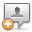KB Home | Advanced Search | News | Ask Question ProSoft Knowledge BaseHome » Categories » Protocols » Modbus

# How do I read/write Modbus binary data?

In a Modbus system, bit-level data is referred to as either "Coils" (read/write bit-level data) or "Input Status" (read only bit-level data.) The Modbus protocol uses Function Codes in commands to specify both the action (read or write) and data object (input coil bits, output coil bits, or input status bits.)

To read Input Status bit-level data, use Function Code 2.

To read Coil bit-level data, use Function Code 1.

To write Coil bit-level data, use Function Code 5 to write a single Coil (bit), or Function Code 15 to write multiple Coils (bits).

For all ProSoft MCM solutions, be sure to use bit-level addresses to the module's internal database for any bit-level Modbus Commands. To find the bit level address for any bit in the module's internal database, use the formula: Register number times 16, plus the bit position number (plus 1 if you are telling a Modbus Master what address to poll when the MCM is a Slave.)

This assumes the first internal database register is Register Address 0 and the lowest order bit of any register is Bit 0.

So, for instance, if you want to write the value of Bit 10 of module database register address 100, the calculation would be:

100 * 16 = 1600 + 10 = 1610 as the Internal Address to use in the bit-level command if the MCM is the Modbus Master, or...

100 * 16 = 1600 + 10 + 1 = 1611, if the MCM is a Slave to a remote Modbus Master.

Custom Fields
Attachments (0)Comments